Ordinary Differential Equation (ODE) Solvers Written in R Using S4 Classes

Show physics, math and engineering students how an ODE solver is made and how effective R classes can be for the construction of the equations that describe natural phenomena. Inspiration for this work comes from the book on "Computer Simulations in Physics" by Harvey Gould, Jan Tobochnik, and Wolfgang Christian. Book link: < http://www.compadre.org/osp/items/detail.cfm?ID=7375>.

rODE

The goal of rODE is to explore R and its S4 classes and its differences with Java and Python classes while exploring physics simulations by solving ordinary differential equations (ODE).

Motivation

This is not your typical black-box ODE solver. You really have to develop your ODE algorithm using any of the ODE solvers available in the package. The objective is learning while coding R, understanding the physics and using the math.

rODE has been inspired on the extraordinary physics library for computer simulations OpenSourcePhyisics. Take a look at it at http://opensourcephysics.org. I highly recommend the book An Introduction to Computer Simulation Methods: Applications To Physical Systems, (Gould, Tobochnik, and Christian, 2017). It has helped me a lot in understanding the physics behind ordinary differential equations. The book briliantly combines code, algorithms, math and physics.

Additionally I have consulted these sources during the developing of the package rODE:

• The beatiful introduction to computers and numerical methods for petroleum engineering "Using the Computer to Solve Petroleum Engineering Problems" by Melvin Nobles", (Nobles, 1974)
• Some examples and analytical solutions were borrowed from "Numerical Solution of Ordinary Differential Equations", (Atkinson, Han, and Stewart, 2009).
• The paper "Numerical Reservoir Simulation Using an Ordinary-Differential-Equations Integrator", (Sincovec, 1975).
• The thesis "Numerical Methods For Solution of Diﬀerential Equations", (Ritschel, 2013).
• The paper "On Dormand-Prince Method" where I could learn about the Dormand-Prince ODE solver, (Kimura, 2009).
• The paper "A Family of Embedded Runge-Kutta formulae", (Dormand and Prince, 1980), where you can see the derivation of the ODE solver RK-45.
• The paper on solving ODEs in R (Soetaert, Petzoldt, and Setzer, 2010).
• The paper "Behind and beyond the Matlab ODE suite" (Ashino, Nagase, and Vaillancourt, 2000).

ODE solvers in this package

The ODE solvers implemented in R so far:

• Euler
• Euler-Richardson
• Verlet
• RK4
• RK45, Dormand-Prince45

Installation

You can install the latest development version of rODE from github with:

Or the stable version from CRAN:

Examples

Example scripts are located under the folder examples inside the package.

These examples make use of a parent class containing a customized rate calculation as well as the step and startup method. The methods that you would commonly find in the base script or parent class are:

• getRate()
• getState()
• step() or doStep()
• setStepSize()
• init(), which is not the same as the S4 class initialize method
• initialize(), and
• the constructor

These methods are defined in the virtual classes ODE and ODESolver.

Two other classes that serve as definition classes for the ODE solvers are: AbstractODESolver and ODEAdaptiveSolver.

For instance, the application KeplerApp.R needs the class Kepler located in the Kepler.R script, which is called with planet <- Kepler(r, v), an ODE object. The solver for the same application is RK45 called with solver <- RK45(planet), where planet is a previuously declared ODE object. Since RK45 is an ODE solver, the script RK45.R will be located in the folder ./R in the package.

Vignettes

The vignettes contain examples of the use of the various ODE solvers.

For instance, the notebook Comparison and Kepler use the ODE solver RK45; FallingParticle and Planet use the Euler solver; Pendulum makes use of EulerRichardson; Planet of Euler, Projectile; Reaction of RK4, and KeplerEnergy uses the ODE solver Verlet.

Tests

There are tests for the core ODE solver classes under tests/testthat, as well as additional tests for the examples themselves.

Test this folder

The tests for the examples are two: one for the base/parent classes such as Kepler or Planet or Projectile; this test runner is called run_tests_this_folder.R.

For the applications there is another runner (run_test_applications.R) that opens each of the applications as request for a return value. If the hard coded value is not returned, the test will fail. This ensures that any minor change in the core solver classes do not have any impact on the application solutions, and if there is, it must be explained.

Tests all the application examples

You can test all applications under the examples folder by running the script run_test_applications.R. The way it works is by getting the list of all applications by filtering those ending with App. Then removes the extension .R from each app and starts looping to call each of the applications with do.call. A list contains the expected results that are compared against the result coming out from the call to the R application.

Applications

• ComparisonRK45App
• FallingParticleODE
• KeplerApp
• KeplerEnergyApp
• LogisticApp
• PendulumApp
• PlanetApp
• ProjectileApp
• ReactionApp
• RigidBodyNXFApp
• SHOApp
• SpringRK4App
• VanderpolApp
• VanderpolMuTimeControlApp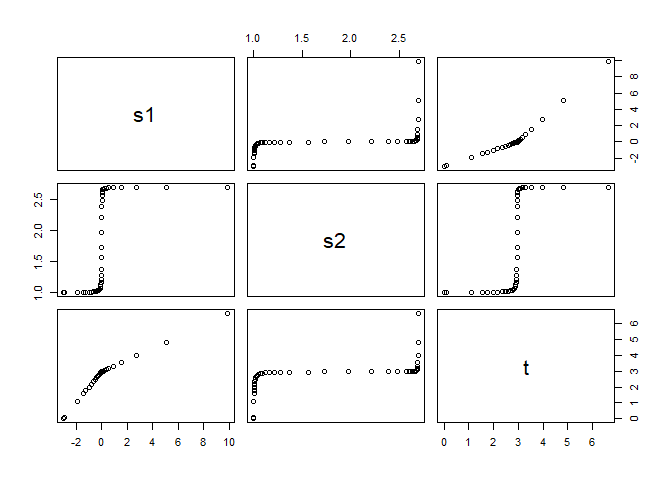ComparisonRK45App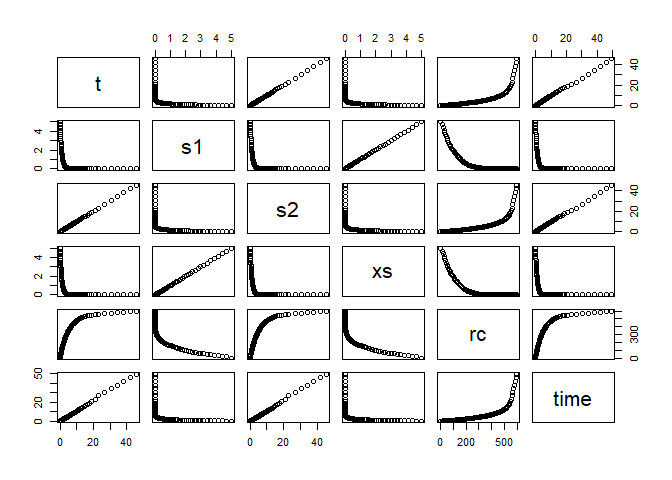FallingParticleODE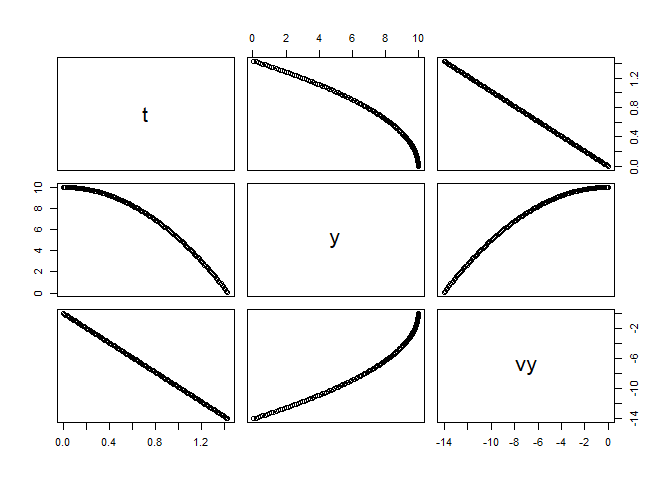KeplerApp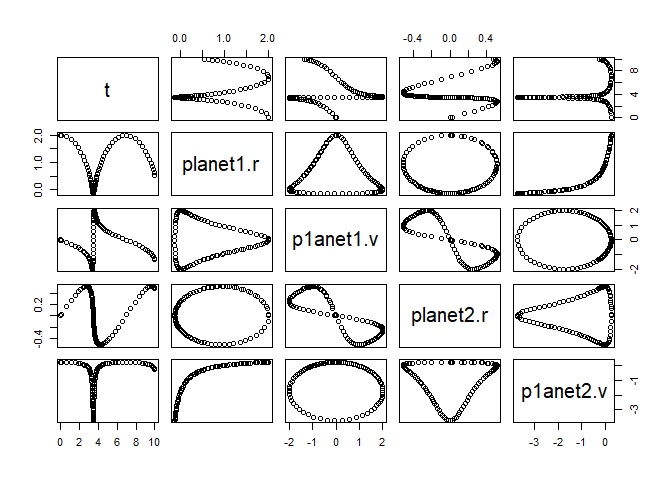KeplerEnergyApp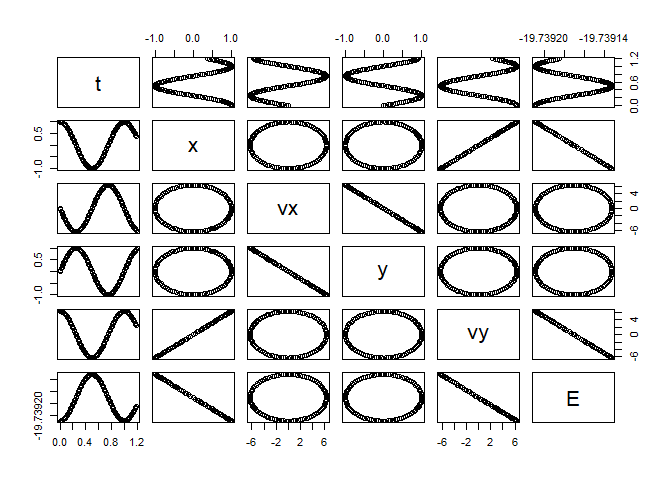LogisticApp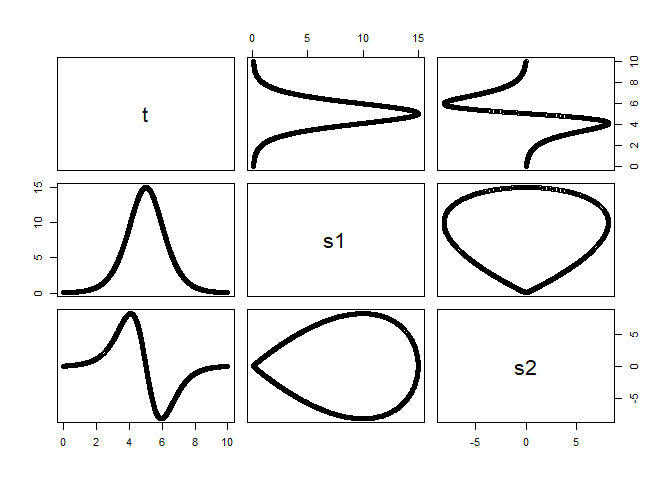PendulumApp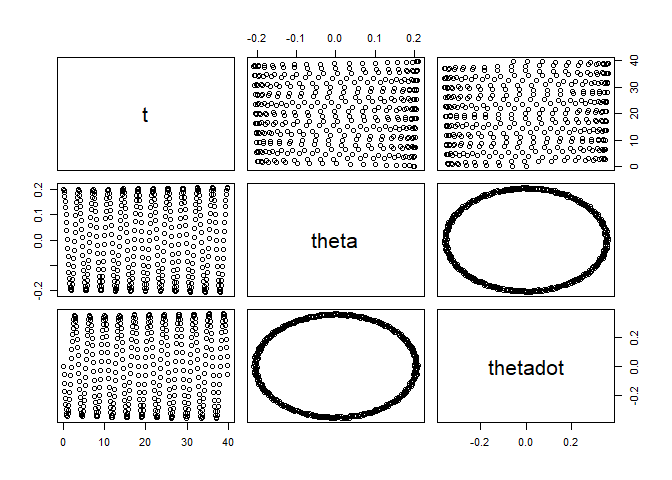PlanetApp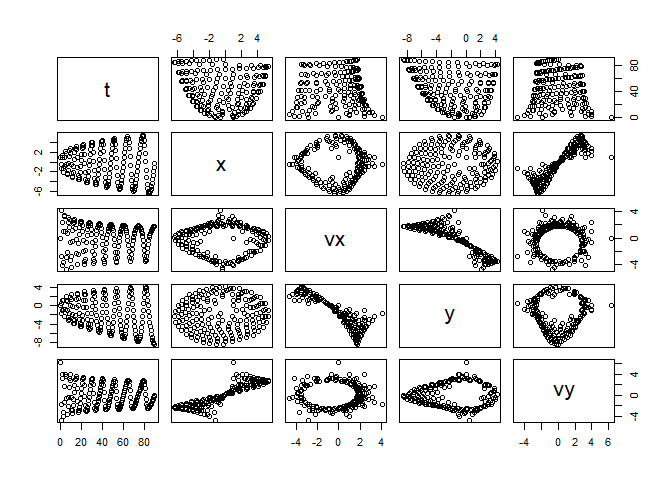ProjectileApp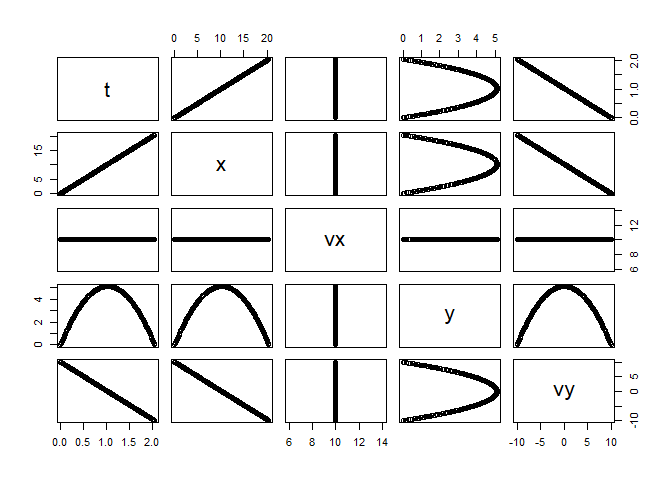ReactionApp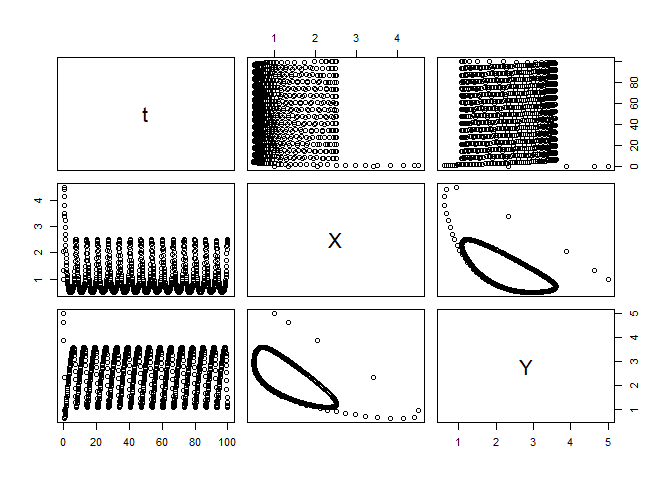RigidBodyNXFApp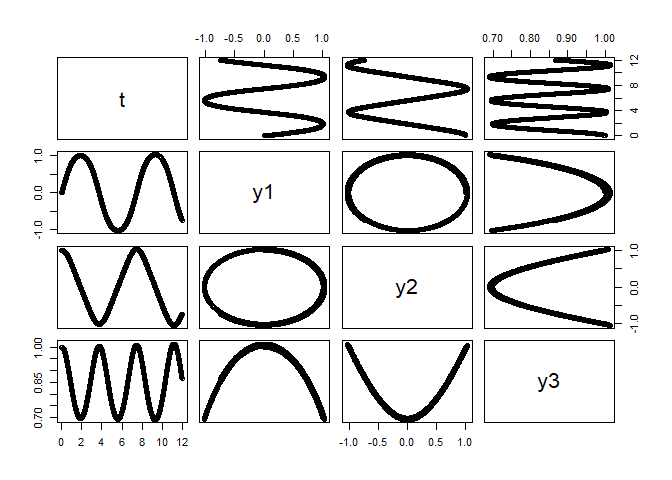SHOApp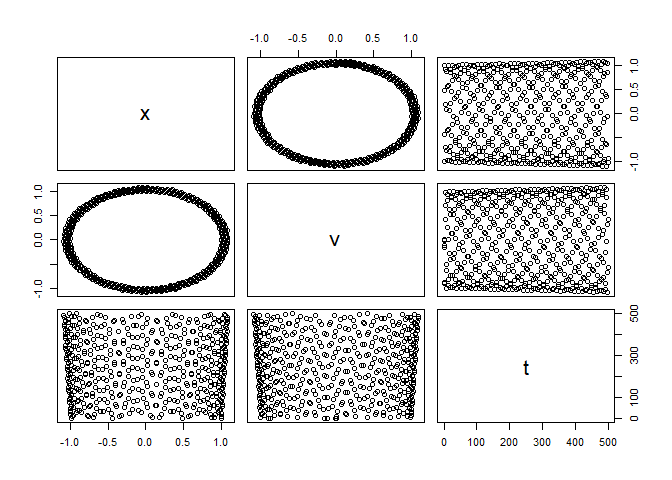SpringRK4App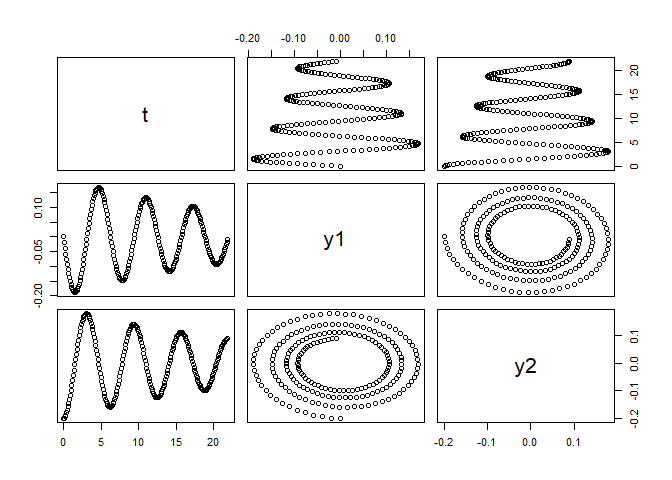VanderpolApp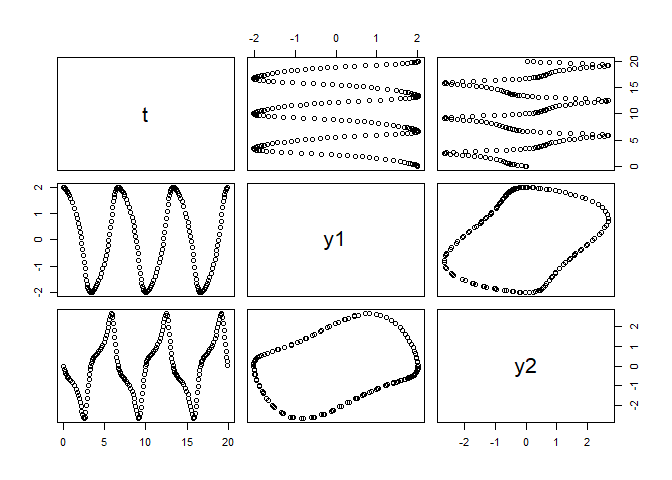VanderpolMuTimeControlApp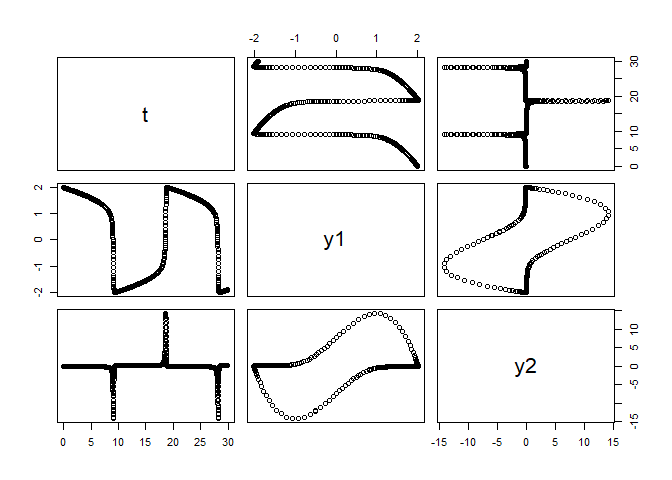References

The following books and papers were consulted during the development of this package:

 R. Ashino, M. Nagase and R. Vaillancourt. "Behind and beyond the Matlab ODE suite". In: Computers & Mathematics with Applications 40.4-5 (Aug. 2000), pp. 491-512. DOI: 10.1016/s0898-1221(00)00175-9.

 K. Atkinson, W. Han and D. E. Stewart. Numerical Solution of Ordinary Differential Equations. Wiley, 2009. ISBN: 978-0-470-04294-6.

 J. R. Dormand and P. J. Prince. "A family of embedded Runge-Kutta formulae". In: Journal of computational and applied mathematics 6.1 (Mar. 1980), pp. 19-26. DOI: 10.1016/0771-050x(80)90013-3.

 H. Gould, J. Tobochnik and W. Christian. An Introduction to Computer Simulation Methods: Applications To Physical Systems. CreateSpace Independent Publishing Platform, 2017. ISBN: 978-1974427475.

 T. Kimura. "On dormand-prince method". In: Retrieved April 27 (2009), p. 2014. <URL: http://depa.fquim.unam.mx/amyd/archivero/DormandPrince_19856.pdf>.

 M. A. Nobles. Using the Computer to Solve Petroleum Engineering Problems. Gulf Publishing Co, 1974. ISBN: 978-0872018860.

 T. Ritschel. "Numerical Methods For Solution of Di<U+FB00>erential Equations". Cand. thesis. DTU supervisor: John Bagterp Jørgensen, [email protected], DTU Compute. Technical University of Denmark, Department of Applied Mathematics and Computer Science, 2013, p. 224. <URL: http://www.compute.dtu.dk/English.aspx>.

 R. Sincovec. "Numerical Reservoir Simulation Using an Ordinary-Differential-Equations Integrator". In: Society of Petroleum Engineers Journal 15.03 (Jun. 1975), pp. 255-264. DOI: 10.2118/5104-pa.

 K. Soetaert, T. Petzoldt and R. W. Setzer. "Solving differential equations in R: package deSolve". In: Journal of Statistical Software 33 (2010).

Reference manual

install.packages("rODE")

0.99.6 by Alfonso R. Reyes, 2 years ago

https://github.com/f0nzie/rODE

Browse source code at https://github.com/cran/rODE

Authors: Alfonso R. Reyes [aut, cre]

Documentation:   PDF Manual

Task views: Differential Equations